# S-99: Ninety-Nine Scala Problems

These are an adaptation of the Ninety-Nine Prolog Problems written by Werner Hett at the Berne University of Applied Sciences in Berne, Switzerland. I (Phil Gold) have altered them to be more amenable to programming in Scala. Feedback is appreciated, particularly on anything marked TODO.

The problems have different levels of difficulty. Those marked with a single asterisk (*) are easy. If you have successfully solved the preceeding problems you should be able to solve them within a few (say 15) minutes. Problems marked with two asterisks (**) are of intermediate difficulty. If you are a skilled Scala programmer it shouldn't take you more than 30-90 minutes to solve them. Problems marked with three asterisks (***) are more difficult. You may need more time (i.e. a few hours or more) to find a good solution. The difficulties were all assigned for the Prolog problems, but the Scala versions seem to be of roughly similar difficulty.

Your goal should be to find the most elegant solution of the given problems. Efficiency is important, but clarity is even more crucial. Some of the (easy) problems can be trivially solved using built-in functions. However, in these cases, you learn more if you try to find your own solution.

Solutions are available by clicking on the link at the beginning of the problem description.

[I don't have example solutions to all of the problems yet. I'm working on getting them all done, but in the meantime, contributed solutions, particularly from seasoned Scala programmers would be appreciated. If you feel a particular problem can be solved in a better manner than I did, please let me know that, too. <PMG>]

## Working with lists

In Scala, lists are objects of type `List[A]`, where A can be any type. Lists are effective for many recursive algorithms, because it's easy to add elements to the head of a list, and to get the tail of the list, which is everything but the first element.

The solutions to the problems in this section will be in objects named after the problems (P01, P02, etc.). You can compile the source files with `scalac` and thereafter use `import` to bring the functions into scope. Some of the problems can be solved easily by using imported solutions to previous problems.

In many cases, there's more than one reasonable approach. The files linked here may include multiple solutions, with all but one commented out. They'll also indicate whether there's a builtin method in Scala that accomplishes the task.

P01 (*) Find the last element of a list.
Example:
```scala> last(List(1, 1, 2, 3, 5, 8))
res0: Int = 8```
P02 (*) Find the last but one element of a list.
Example:
```scala> penultimate(List(1, 1, 2, 3, 5, 8))
res0: Int = 5```
P03 (*) Find the Kth element of a list.
By convention, the first element in the list is element 0.

Example:

```scala> nth(2, List(1, 1, 2, 3, 5, 8))
res0: Int = 2```
P04 (*) Find the number of elements of a list.
Example:
```scala> length(List(1, 1, 2, 3, 5, 8))
res0: Int = 6```
P05 (*) Reverse a list.
Example:
```scala> reverse(List(1, 1, 2, 3, 5, 8))
res0: List[Int] = List(8, 5, 3, 2, 1, 1)```
P06 (*) Find out whether a list is a palindrome.
Example:
```scala> isPalindrome(List(1, 2, 3, 2, 1))
res0: Boolean = true```
P07 (**) Flatten a nested list structure.
Example:
```scala> flatten(List(List(1, 1), 2, List(3, List(5, 8))))
res0: List[Any] = List(1, 1, 2, 3, 5, 8)```
P08 (**) Eliminate consecutive duplicates of list elements.
If a list contains repeated elements they should be replaced with a single copy of the element. The order of the elements should not be changed.

Example:

```scala> compress(List('a, 'a, 'a, 'a, 'b, 'c, 'c, 'a, 'a, 'd, 'e, 'e, 'e, 'e))
res0: List[Symbol] = List('a, 'b, 'c, 'a, 'd, 'e)```
P09 (**) Pack consecutive duplicates of list elements into sublists.
If a list contains repeated elements they should be placed in separate sublists.

Example:

```scala> pack(List('a, 'a, 'a, 'a, 'b, 'c, 'c, 'a, 'a, 'd, 'e, 'e, 'e, 'e))
res0: List[List[Symbol]] = List(List('a, 'a, 'a, 'a), List('b), List('c, 'c), List('a, 'a), List('d), List('e, 'e, 'e, 'e))```
P10 (*) Run-length encoding of a list.
Use the result of problem P09 to implement the so-called run-length encoding data compression method. Consecutive duplicates of elements are encoded as tuples ```(N, E)``` where N is the number of duplicates of the element E.

Example:

```scala> encode(List('a, 'a, 'a, 'a, 'b, 'c, 'c, 'a, 'a, 'd, 'e, 'e, 'e, 'e))
res0: List[(Int, Symbol)] = List((4,'a), (1,'b), (2,'c), (2,'a), (1,'d), (4,'e))```
P11 (*) Modified run-length encoding.
Modify the result of problem P10 in such a way that if an element has no duplicates it is simply copied into the result list. Only elements with duplicates are transferred as `(N, E)` terms.

Example:

```scala> encodeModified(List('a, 'a, 'a, 'a, 'b, 'c, 'c, 'a, 'a, 'd, 'e, 'e, 'e, 'e))
res0: List[Any] = List((4,'a), 'b, (2,'c), (2,'a), 'd, (4,'e))```
P12 (**) Decode a run-length encoded list.
Given a run-length code list generated as specified in problem P10, construct its uncompressed version.

Example:

```scala> decode(List((4, 'a), (1, 'b), (2, 'c), (2, 'a), (1, 'd), (4, 'e)))
res0: List[Symbol] = List('a, 'a, 'a, 'a, 'b, 'c, 'c, 'a, 'a, 'd, 'e, 'e, 'e, 'e)```
P13 (**) Run-length encoding of a list (direct solution).
Implement the so-called run-length encoding data compression method directly. I.e. don't use other methods you've written (like P09's `pack`); do all the work directly.

Example:

```scala> encodeDirect(List('a, 'a, 'a, 'a, 'b, 'c, 'c, 'a, 'a, 'd, 'e, 'e, 'e, 'e))
res0: List[(Int, Symbol)] = List((4,'a), (1,'b), (2,'c), (2,'a), (1,'d), (4,'e))```
P14 (*) Duplicate the elements of a list.
Example:
```scala> duplicate(List('a, 'b, 'c, 'c, 'd))
res0: List[Symbol] = List('a, 'a, 'b, 'b, 'c, 'c, 'c, 'c, 'd, 'd)```
P15 (**) Duplicate the elements of a list a given number of times.
Example:
```scala> duplicateN(3, List('a, 'b, 'c, 'c, 'd))
res0: List[Symbol] = List('a, 'a, 'a, 'b, 'b, 'b, 'c, 'c, 'c, 'c, 'c, 'c, 'd, 'd, 'd)```
P16 (**) Drop every Nth element from a list.
Example:
```scala> drop(3, List('a, 'b, 'c, 'd, 'e, 'f, 'g, 'h, 'i, 'j, 'k))
res0: List[Symbol] = List('a, 'b, 'd, 'e, 'g, 'h, 'j, 'k)```
P17 (*) Split a list into two parts.
The length of the first part is given. Use a Tuple for your result.

Example:

```scala> split(3, List('a, 'b, 'c, 'd, 'e, 'f, 'g, 'h, 'i, 'j, 'k))
res0: (List[Symbol], List[Symbol]) = (List('a, 'b, 'c),List('d, 'e, 'f, 'g, 'h, 'i, 'j, 'k))```
P18 (**) Extract a slice from a list.
Given two indices, I and K, the slice is the list containing the elements from and including the Ith element up to but not including the Kth element of the original list. Start counting the elements with 0.

Example:

```scala> slice(3, 7, List('a, 'b, 'c, 'd, 'e, 'f, 'g, 'h, 'i, 'j, 'k))
res0: List[Symbol] = List('d, 'e, 'f, 'g)```
P19 (**) Rotate a list N places to the left.
Examples:
```scala> rotate(3, List('a, 'b, 'c, 'd, 'e, 'f, 'g, 'h, 'i, 'j, 'k))
res0: List[Symbol] = List('d, 'e, 'f, 'g, 'h, 'i, 'j, 'k, 'a, 'b, 'c)

scala> rotate(-2, List('a, 'b, 'c, 'd, 'e, 'f, 'g, 'h, 'i, 'j, 'k))
res1: List[Symbol] = List('j, 'k, 'a, 'b, 'c, 'd, 'e, 'f, 'g, 'h, 'i)```
P20 (*) Remove the Kth element from a list.
Return the list and the removed element in a Tuple. Elements are numbered from 0.

Example:

```scala> removeAt(1, List('a, 'b, 'c, 'd))
res0: (List[Symbol], Symbol) = (List('a, 'c, 'd),'b)```
P21 (*) Insert an element at a given position into a list.
Example:
```scala> insertAt('new, 1, List('a, 'b, 'c, 'd))
res0: List[Symbol] = List('a, 'new, 'b, 'c, 'd)```
P22 (*) Create a list containing all integers within a given range.
Example:
```scala> range(4, 9)
res0: List[Int] = List(4, 5, 6, 7, 8, 9)```
P23 (**) Extract a given number of randomly selected elements from a list.
Example:
```scala> randomSelect(3, List('a, 'b, 'c, 'd, 'f, 'g, 'h))
res0: List[Symbol] = List('e, 'd, 'a)```

Hint: Use the solution to problem P20

P24 (*) Lotto: Draw N different random numbers from the set 1..M.
Example:
```scala> lotto(6, 49)
res0: List[Int] = List(23, 1, 17, 33, 21, 37)```
P25 (*) Generate a random permutation of the elements of a list.
Hint: Use the solution of problem P23.

Example:

```scala> randomPermute(List('a, 'b, 'c, 'd, 'e, 'f))
res0: List[Symbol] = List('b, 'a, 'd, 'c, 'e, 'f)```
P26 (**) Generate the combinations of K distinct objects chosen from the N elements of a list.
In how many ways can a committee of 3 be chosen from a group of 12 people? We all know that there are `C(12,3) = 220` possibilities (`C(N,K)` denotes the well-known binomial coefficient). For pure mathematicians, this result may be great. But we want to really generate all the possibilities.

Example:

```scala> combinations(3, List('a, 'b, 'c, 'd, 'e, 'f))
res0: List[List[Symbol]] = List(List('a, 'b, 'c), List('a, 'b, 'd), List('a, 'b, 'e), ...```
P27 (**) Group the elements of a set into disjoint subsets.
a) In how many ways can a group of 9 people work in 3 disjoint subgroups of 2, 3 and 4 persons? Write a function that generates all the possibilities.

Example:

```scala> group3(List("Aldo", "Beat", "Carla", "David", "Evi", "Flip", "Gary", "Hugo", "Ida"))
res0: List[List[List[String]]] = List(List(List(Aldo, Beat), List(Carla, David, Evi), List(Flip, Gary, Hugo, Ida)), ...```

b) Generalize the above predicate in a way that we can specify a list of group sizes and the predicate will return a list of groups.

Example:

```scala> group(List(2, 2, 5), List("Aldo", "Beat", "Carla", "David", "Evi", "Flip", "Gary", "Hugo", "Ida"))
res0: List[List[List[String]]] = List(List(List(Aldo, Beat), List(Carla, David), List(Evi, Flip, Gary, Hugo, Ida)), ...```

Note that we do not want permutations of the group members; i.e. ((Aldo, Beat), ...) is the same solution as ((Beat, Aldo), ...). However, we make a difference between ((Aldo, Beat), (Carla, David), ...) and ((Carla, David), (Aldo, Beat), ...).

You may find more about this combinatorial problem in a good book on discrete mathematics under the term "multinomial coefficients".

P28 (**) Sorting a list of lists according to length of sublists.
a) We suppose that a list contains elements that are lists themselves. The objective is to sort the elements of the list according to their length. E.g. short lists first, longer lists later, or vice versa.

Example:

```scala> lsort(List(List('a, 'b, 'c), List('d, 'e), List('f, 'g, 'h), List('d, 'e), List('i, 'j, 'k, 'l), List('m, 'n), List('o)))
res0: List[List[Symbol]] = List(List('o), List('d, 'e), List('d, 'e), List('m, 'n), List('a, 'b, 'c), List('f, 'g, 'h), List('i, 'j, 'k, 'l))```

b) Again, we suppose that a list contains elements that are lists themselves. But this time the objective is to sort the elements according to their length frequency; i.e. in the default, sorting is done ascendingly, lists with rare lengths are placed, others with a more frequent length come later.

Example:

```scala> lsortFreq(List(List('a, 'b, 'c), List('d, 'e), List('f, 'g, 'h), List('d, 'e), List('i, 'j, 'k, 'l), List('m, 'n), List('o)))
res1: List[List[Symbol]] = List(List('i, 'j, 'k, 'l), List('o), List('a, 'b, 'c), List('f, 'g, 'h), List('d, 'e), List('d, 'e), List('m, 'n))```

Note that in the above example, the first two lists in the result have length 4 and 1 and both lengths appear just once. The third and fourth lists have length 3 and there are two list of this length. Finally, the last three lists have length 2. This is the most frequent length.

## Arithmetic

For the next section, we're going to take a different tack with the solutions. We'll declare a new class, `S99Int`, and an implicit conversion from regular `Int`s. The arithmetic1 file contains the starting definitions for this section. Each individual solution will show the relevant additions to the `S99Int` class. The full class will be given at the end of the section.

P31 (**) Determine whether a given integer number is prime.
```scala> 7.isPrime
res0: Boolean = true```
P32 (**) Determine the greatest common divisor of two positive integer numbers.
Use Euclid's algorithm.
```scala> gcd(36, 63)
res0: Int = 9```
P33 (*) Determine whether two positive integer numbers are coprime.
Two numbers are coprime if their greatest common divisor equals 1.
```scala> 35.isCoprimeTo(64)
res0: Boolean = true```
P34 (**) Calculate Euler's totient function `phi(m)`.
Euler's so-called totient function `phi(m)` is defined as the number of positive integers r (1 <= r <= m) that are coprime to m.
```scala> 10.totient
res0: Int = 4```
P35 (**) Determine the prime factors of a given positive integer.
Construct a flat list containing the prime factors in ascending order.
```scala> 315.primeFactors
res0: List[Int] = List(3, 3, 5, 7)```
P36 (**) Determine the prime factors of a given positive integer (2).
Construct a list containing the prime factors and their multiplicity.
```scala> 315.primeFactorMultiplicity
res0: List[(Int, Int)] = List((3,2), (5,1), (7,1))```

Alternately, use a Map for the result.

```scala> 315.primeFactorMultiplicity
res0: Map[Int,Int] = Map(3 -> 2, 5 -> 1, 7 -> 1)```
P37 (**) Calculate Euler's totient function phi(m) (improved).
See problem P34 for the definition of Euler's totient function. If the list of the prime factors of a number m is known in the form of problem P36 then the function `phi(m>)` can be efficiently calculated as follows: Let [[p1, m1], [p2, m2], [p3, m3], ...] be the list of prime factors (and their multiplicities) of a given number m. Then `phi(m)` can be calculated with the following formula:

phi(m) = (p1-1)*p1(m1-1) * (p2-1)*p2(m2-1) * (p3-1)*p3(m3-1) * ...

Note that ab stands for the bth power of a.

P38 (*) Compare the two methods of calculating Euler's totient function.
Use the solutions of problems P34 and P37 to compare the algorithms. Try to calculate phi(10090) as an example.

P39 (*) A list of prime numbers.
Given a range of integers by its lower and upper limit, construct a list of all prime numbers in that range.
```scala> listPrimesinRange(7 to 31)
res0: List[Int] = List(7, 11, 13, 17, 19, 23, 29, 31)```
P40 (**) Goldbach's conjecture.
Goldbach's conjecture says that every positive even number greater than 2 is the sum of two prime numbers. E.g. 28 = 5 + 23. It is one of the most famous facts in number theory that has not been proved to be correct in the general case. It has been numerically confirmed up to very large numbers (much larger than Scala's Int can represent). Write a function to find the two prime numbers that sum up to a given even integer.
```scala> 28.goldbach
res0: (Int, Int) = (5,23)```
P41 (**) A list of Goldbach compositions.
Given a range of integers by its lower and upper limit, print a list of all even numbers and their Goldbach composition.
```scala> printGoldbachList(9 to 20)
10 = 3 + 7
12 = 5 + 7
14 = 3 + 11
16 = 3 + 13
18 = 5 + 13
20 = 3 + 17```

In most cases, if an even number is written as the sum of two prime numbers, one of them is very small. Very rarely, the primes are both bigger than, say, 50. Try to find out how many such cases there are in the range 2..3000.

Example (minimum value of 50 for the primes):

```scala> printGoldbachListLimited(1 to 2000, 50)
992 = 73 + 919
1382 = 61 + 1321
1856 = 67 + 1789
1928 = 61 + 1867```

The file containing the full class for this section is arithmetic.scala.

## Logic and Codes

As in the previous section, we will start with a skeleton file, logic1.scala, and add code to it for each problem. The difference here is that the file starts out almost empty.

P46 (**) Truth tables for logical expressions.
Define functions `and`, `or`, `nand`, `nor`, `xor`, `impl`, and `equ` (for logical equivalence) which return true or false according to the result of their respective operations; e.g. `and(A, B)` is true if and only if both A and B are true.
```scala> and(true, true)
res0: Boolean = true

scala> xor(true. true)
res1: Boolean = false```

A logical expression in two variables can then be written as an function of two variables, e.g: ```(a: Boolean, b: Boolean) => and(or(a, b), nand(a, b))```

Now, write a function called `table2` which prints the truth table of a given logical expression in two variables.

```scala> table2((a: Boolean, b: Boolean) => and(a, or(a, b)))
A     B     result
true  true  true
true  false true
false true  false
false false false```
P47 (*) Truth tables for logical expressions (2).
Continue problem P46 by redefining `and`, `or`, etc as operators. (i.e. make them methods of a new class with an implicit conversion from Boolean.) `not` will have to be left as a object method.
```scala> table2((a: Boolean, b: Boolean) => a and (a or not(b)))
A     B     result
true  true  true
true  false true
false true  false
false false false```
P48 (**) Truth tables for logical expressions (3).
Omitted for now.

P49 (**) Gray code.
An n-bit Gray code is a sequence of n-bit strings constructed according to certain rules. For example,
n = 1: C(1) = ("0", "1").
n = 2: C(2) = ("00", "01", "11", "10").
n = 3: C(3) = ("000", "001", "011", "010", "110", "111", "101", "100").

Find out the construction rules and write a function to generate Gray codes.

```scala> gray(3)
res0 List[String] = List(000, 001, 011, 010, 110, 111, 101, 100)```

See if you can use memoization to make the function more efficient.

P50 (***) Huffman code.
First of all, consult a good book on discrete mathematics or algorithms for a detailed description of Huffman codes!

We suppose a set of symbols with their frequencies, given as a list of `(S, F)` Tuples. E.g. ```(("a", 45), ("b", 13), ("c", 12), ("d", 16), ("e", 9), ("f", 5))```. Our objective is to construct a list of `(S, C)` Tuples, where C is the Huffman code word for the symbol S.

```scala> huffman(List(("a", 45), ("b", 13), ("c", 12), ("d", 16), ("e", 9), ("f", 5)))
res0: List[String, String] = List((a,0), (b,101), (c,100), (d,111), (e,1101), (f,1100))```

## Binary Trees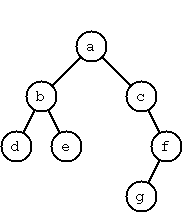A binary tree is either empty or it is composed of a root element and two successors, which are binary trees themselves.

We shall use the following classes to represent binary trees. (Also available in tree1.scala.) An End is equivalent to an empty tree. A Branch has a value, and two descendant trees. The `toString` functions are relatively arbitrary, but they yield a more compact output than Scala's default. Putting a plus in front of the `T` makes the class covariant; it will be able to hold subtypes of whatever type it's created for. (This is important so that `End` can be a singleton object; as a singleton, it must have a specific type, so we give it type `Nothing`, which is a subtype of every other type.)

```sealed abstract class Tree[+T]
case class Node[+T](value: T, left: Tree[T], right: Tree[T]) extends Tree[T] {
override def toString = "T(" + value.toString + " " + left.toString + " " + right.toString + ")"
}
case object End extends Tree[Nothing] {
override def toString = "."
}
object Node {
def apply[T](value: T): Node[T] = Node(value, End, End)
}```

The example tree on the right is given by

```Node('a',
Node('b', Node('d'), Node('e')),
Node('c', End, Node('f', Node('g'), End)))```

A tree with only a root node would be `Node('a')` and an empty tree would be `End`.

Throughout this section, we will be adding methods to the classes above, mostly to `Tree`.

P54 Omitted; our tree representation will only allow well-formed trees.
Score one for static typing.

P55 (**) Construct completely balanced binary trees.
In a completely balanced binary tree, the following property holds for every node: The number of nodes in its left subtree and the number of nodes in its right subtree are almost equal, which means their difference is not greater than one.

Define an object named `Tree`. Write a function `Tree.cBalanced` to construct completely balanced binary trees for a given number of nodes. The function should generate all solutions. The function should take as parameters the number of nodes and a single value to put in all of them.

```scala> Tree.cBalanced(4, "x")
res0: List(Node[String]) = List(T(x T(x . .) T(x . T(x . .))), T(x T(x . .) T(x T(x . .) .)), ...```
P56 (**) Symmetric binary trees.
Let us call a binary tree symmetric if you can draw a vertical line through the root node and then the right subtree is the mirror image of the left subtree. Add an `isSymmetric` method to the Tree class to check whether a given binary tree is symmetric. Hint: Write an `isMirrorOf` method first to check whether one tree is the mirror image of another. We are only interested in the structure, not in the contents of the nodes.
```scala> Node('a', Node('b'), Node('c')).isSymmetric
res0: Boolean = true```
P57 (**) Binary search trees (dictionaries).
Write a function to add an element to a binary search tree.
```scala> End.addValue(2)
res0: Node[Int] = T(2 . .)

res1: Node[Int] = T(2 . T(3 . .))

res2: Node[Int] = T(2 T(0 . .) T(3 . .))```

Hint: The abstract definition of `addValue` in `Tree` should be ```def addValue[U >: T <% Ordered[U]](x: U): Tree[U]```. The `>: T` is because `addValue`'s parameters need to be contravariant in `T`. (Conceptually, we're adding nodes above existing nodes. In order for the subnodes to be of type `T` or any subtype, the upper nodes must be of type `T` or any supertype.) The `<% Ordered[U]` allows us to use the `<` operator on the values in the tree.

Use that function to construct a binary tree from a list of integers.

```scala> Tree.fromList(List(3, 2, 5, 7, 1))
res3: Node[Int] = T(3 T(2 T(1 . .) .) T(5 . T(7 . .)))```

Finally, use that function to test your solution to P56.

```scala> Tree.fromList(List(5, 3, 18, 1, 4, 12, 21)).isSymmetric
res4: Boolean = true

scala> Tree.fromList(List(3, 2, 5, 7, 4)).isSymmetric
res5: Boolean = false```
Apply the generate-and-test paradigm to construct all symmetric, completely balanced binary trees with a given number of nodes.
```scala> Tree.symmetricBalancedTrees(5, "x")
res0: List[Node[String]] = List(T(x T(x . T(x . .)) T(x T(x . .) .)), T(x T(x T(x . .) .) T(x . T(x . .))))```
P59 (**) Construct height-balanced binary trees.
In a height-balanced binary tree, the following property holds for every node: The height of its left subtree and the height of its right subtree are almost equal, which means their difference is not greater than one.

Write a method `Tree.hbalTrees` to construct height-balanced binary trees for a given height with a supplied value for the nodes. The function should generate all solutions.

```scala> Tree.hbalTrees(3, "x")
res0: List[Node[String]] = List(T(x T(x T(x . .) T(x . .)) T(x T(x . .) T(x . .))), T(x T(x T(x . .) T(x . .)) T(x T(x . .) .)), ...```
P60 (**) Construct height-balanced binary trees with a given number of nodes.
Consider a height-balanced binary tree of height H. What is the maximum number of nodes it can contain? Clearly, MaxN = 2H - 1. However, what is the minimum number MinN? This question is more difficult. Try to find a recursive statement and turn it into a function `minHbalNodes` that takes a height and returns MinN.
```scala> minHbalNodes(3)
res0: Int = 4```

On the other hand, we might ask: what is the maximum height H a height-balanced binary tree with N nodes can have? Write a `maxHbalHeight` function.

```scala> maxHbalHeight(4)
res1: Int = 3```

Now, we can attack the main problem: construct all the height-balanced binary trees with a given nuber of nodes.

```scala> Tree.hbalTreesWithNodes(4, "x")
res2: List[Node[String]] = List(T(x T(x T(x . .) .) T(x . .)), T(x T(x . T(x . .)) T(x . .)), ...```

Find out how many height-balanced trees exist for N = 15.

P61 (*) Count the leaves of a binary tree.
A leaf is a node with no successors. Write a method `leafCount` to count them.
```scala> Node('x', Node('x'), End).leafCount
res0: Int = 1```
61A (*) Collect the leaves of a binary tree in a list.
A leaf is a node with no successors. Write a method `leafList` to collect them in a list.
```scala> Node('a', Node('b'), Node('c', Node('d'), Node('e'))).leafList
res0: List[Char] = List(b, d, e)```
P62 (*) Collect the internal nodes of a binary tree in a list.
An internal node of a binary tree has either one or two non-empty successors. Write a method `internalList` to collect them in a list.
```scala> Node('a', Node('b'), Node('c', Node('d'), Node('e'))).internalList
res0: List[Char] = List(a, c)```
P62B (*) Collect the nodes at a given level in a list.
A node of a binary tree is at level N if the path from the root to the node has length N-1. The root node is at level 1. Write a method `atLevel` to collect all nodes at a given level in a list.
```scala> Node('a', Node('b'), Node('c', Node('d'), Node('e'))).atLevel(2)
res0: List[Char] = List(b, c)```

Using `atLevel` it is easy to construct a method `levelOrder` which creates the level-order sequence of the nodes. However, there are more efficient ways to do that.

P63 (**) Construct a complete binary tree.
A complete binary tree with height H is defined as follows: The levels 1,2,3,...,H-1 contain the maximum number of nodes (i.e 2(i-1) at the level i, note that we start counting the levels from 1 at the root). In level H, which may contain less than the maximum possible number of nodes, all the nodes are "left-adjusted". This means that in a levelorder tree traversal all internal nodes come first, the leaves come second, and empty successors (the `End`s which are not really nodes!) come last.

Particularly, complete binary trees are used as data structures (or addressing schemes) for heaps.

We can assign an address number to each node in a complete binary tree by enumerating the nodes in levelorder, starting at the root with number 1. In doing so, we realize that for every node X with address A the following property holds: The address of X's left and right successors are 2*A and 2*A+1, respectively, supposed the successors do exist. This fact can be used to elegantly construct a complete binary tree structure. Write a method `completeBinaryTree` that takes as parameters the number of nodes and the value to put in each node.

```scala> Tree.completeBinaryTree(6, "x")
res0: Node[String] = T(x T(x T(x . .) T(x . .)) T(x T(x . .) .))```
P64 (**) Layout a binary tree (1).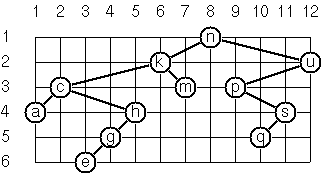As a preparation for drawing a tree, a layout algorithm is required to determine the position of each node in a rectangular grid. Several layout methods are conceivable, one of them is shown in the illustration on the right.

In this layout strategy, the position of a node v is obtained by the following two rules:

• x(v) is equal to the position of the node v in the inorder sequence
• y(v) is equal to the depth of the node v in the tree

In order to store the position of the nodes, we add a new class with the additional information.

```case class PositionedNode[+T](override val value: T, override val left: Tree[T], override val right: Tree[T], x: Int, y: Int) extends Node[T](value, left, right) {
override def toString = "T[" + x.toString + "," + y.toString + "](" + value.toString + " " + left.toString + " " + right.toString + ")"
}```

Write a method `layoutBinaryTree` that turns a tree of normal `Node`s into a tree of `PositionedNode`s.

```scala> Node('a', Node('b', End, Node('c')), Node('d')).layoutBinaryTree
res0: PositionedNode[Char] = T[3,1](a T[1,2](b . T[2,3](c . .)) T[4,2](d . .))```

The tree at right may be constructed with `Tree.fromList(List('n','k','m','c','a','h','g','e','u','p','s','q'))`. Use it to check your code.

P65 (**) Layout a binary tree (2).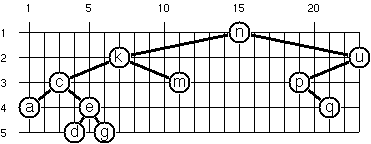An alternative layout method is depicted in the illustration opposite. Find out the rules and write the corresponding method. Hint: On a given level, the horizontal distance between neighboring nodes is constant.

Use the same conventions as in problem P64.

```scala> Node('a', Node('b', End, Node('c')), Node('d')).layoutBinaryTree2
res0: PositionedNode[Char] = T[3,1]('a T[1,2]('b . T[2,3]('c . .)) T[5,2]('d . .))```

The tree at right may be constructed with `Tree.fromList(List('n','k','m','c','a','e','d','g','u','p','q'))`. Use it to check your code.

P66 (***) Layout a binary tree (3).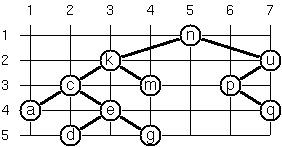Yet another layout strategy is shown in the illustration opposite. The method yields a very compact layout while maintaining a certain symmetry in every node. Find out the rules and write the corresponding method. Hint: Consider the horizontal distance between a node and its successor nodes. How tight can you pack together two subtrees to construct the combined binary tree?

Use the same conventions as in problem P64 and P65. Note: This is a difficult problem. Don't give up too early!

```scala> Node('a', Node('b', End, Node('c')), Node('d')).layoutBinaryTree3
res0: PositionedNode[Char] = T[2,1]('a T[1,2]('b . T[2,3]('c . .)) T[3,2]('d . .))```

Which layout do you like most?

P67 (**) A string representation of binary trees.Somebody represents binary trees as strings of the following type (see example opposite):
`a(b(d,e),c(,f(g,)))`

Write a method which generates this string representation, if the tree is given as usual (in `Node`s and `End`s). Use that method for the `Tree` class's and subclass's `toString` methods. Then write a method (on the `Tree` object) which does this inverse; i.e. given the string representation, construct the tree in the usual form.

For simplicity, suppose the information in the nodes is a single letter and there are no spaces in the string.

```scala> Node('a', Node('b', Node('d'), Node('e')), Node('c', End, Node('f', Node('g'), End))).toString
res0: String = a(b(d,e),c(,f(g,)))

scala> Tree.fromString("a(b(d,e),c(,f(g,)))")
res1: Node[Char] = a(b(d,e),c(,f(g,)))```
P68 (**) Preorder and inorder sequences of binary trees.
We consider binary trees with nodes that are identified by single lower-case letters, as in the example of problem P67.

a) Write methods `preorder` and `inorder` that construct the preorder and inorder sequence of a given binary tree, respectively. The results should be lists, e.g. `List('a','b','d','e','c','f','g')` for the preorder sequence of the example in problem P67.

```scala> Tree.string2Tree("a(b(d,e),c(,f(g,)))").preorder
res0: List[Char] = List(a, b, d, e, c, f, g)

scala> Tree.string2Tree("a(b(d,e),c(,f(g,)))").inorder
res1: List[Char] = List(d, b, e, a, c, g, f)```

b) If both the preorder sequence and the inorder sequence of the nodes of a binary tree are given, then the tree is determined unambiguously. Write a method `preInTree` that does the job.

```scala> Tree.preInTree(List('a', 'b', 'd', 'e', 'c', 'f', 'g'), List('d', 'b', 'e', 'a', 'c', 'g', 'f'))
res2: Node[Char] = a(b(d,e),c(,f(g,)))```

What happens if the same character appears in more than one node? Try, for instance, ```Tree.preInTree(List('a', 'b', 'a'), List('b', 'a', 'a'))```.

P69 (**) Dotstring representation of binary trees.
We consider again binary trees with nodes that are identified by single lower-case letters, as in the example of problem P67. Such a tree can be represented by the preorder sequence of its nodes in which dots (.) are inserted where an empty subtree (`End`) is encountered during the tree traversal. For example, the tree shown in problem P67 is represented as `"abd..e..c.fg..."`. First, try to establish a syntax (BNF or syntax diagrams) and then write two methods, `toDotstring` and `fromDotstring`, which do the conversion in both directions.
```scala> Tree.string2Tree("a(b(d,e),c(,f(g,)))").toDotstring
res0: String = abd..e..c.fg...

scala> Tree.fromDotstring("abd..e..c.fg...")
res1: Node[Char] = a(b(d,e),c(,f(g,)))```

The file containing the full class definitions for this section is tree.scala.

## Multiway Trees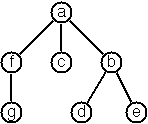A multiway tree is composed of a root element and a (possibly empty) set of successors which are multiway trees themselves. A multiway tree is never empty. The set of successor trees is sometimes called a forest.

The code to represent these is somewhat simpler than the code for binary trees, partly because we don't separate classes for nodes and terminators, and partly because we don't need the restriction that the value type be ordered.

```case class MTree[+T](value: T, children: List[MTree[T]]) {
def this(value: T) = this(value, List())
override def toString = "M(" + value.toString + " {" + children.map(_.toString).mkString(",") + "})"
}

object MTree {
def apply[T](value: T) = new MTree(value, List())
def apply[T](value: T, children: List[MTree[T]]) = new MTree(value, children)
}```

The example tree is, thus:

`MTree('a', List(MTree('f', List(MTree('g'))), MTree('c'), MTree('b', List(MTree('d'), MTree('e')))))`

The starting code skeleton for this section is mtree1.scala.

P70B Omitted; we can only create well-formed trees.

P70C (*) Count the nodes of a multiway tree.
Write a method `nodeCount` which counts the nodes of a given multiway tree.
```scala> MTree('a', List(MTree('f'))).nodeCount
res0: Int = 2```
P70 (**) Tree construction from a node string.We suppose that the nodes of a multiway tree contain single characters. In the depth-first order sequence of its nodes, a special character ^ has been inserted whenever, during the tree traversal, the move is a backtrack to the previous level.

By this rule, the tree in the figure opposite is represented as:

`afg^^c^bd^e^^^`

Define the syntax of the string and write a function `string2MTree` to construct an `MTree` from a `String`. Make the function an implicit conversion from `String`. Write the reverse function, and make it the toString method of MTree.

```scala> MTree('a', List(MTree('f', List(MTree('g'))), MTree('c'), MTree('b', List(MTree('d'), MTree('e'))))).toString
res0: String = afg^^c^bd^e^^^```
P71 (*) Determine the internal path length of a tree.
We define the internal path length of a multiway tree as the total sum of the path lengths from the root to all nodes of the tree. By this definition, the tree in the figure of problem P70 has an internal path length of 9. Write a method `internalPathLength` to return that sum.
```scala> "afg^^c^bd^e^^^".internalPathLength
res0: Int = 9```
P72 (*) Construct the postorder sequence of the tree nodes.
Write a method `postorder` which constructs the postorder sequence of the nodes of a multiway tree. The result should be a `List`.
```scala> "afg^^c^bd^e^^^".postorder
res0: List[Char] = List(g, f, c, d, e, b, a)```
P73 (**) Lisp-like tree representation.
There is a particular notation for multiway trees in Lisp. Lisp is a prominent functional programming language. In Lisp almost everything is a list.

Our example tree would be represented in Lisp as ```(a (f g) c (b d e))```. The following pictures give some more examples.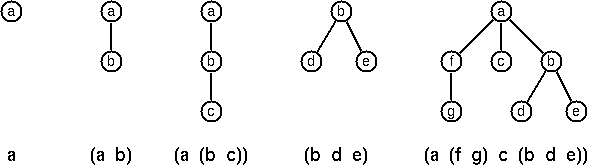Note that in the "lispy" notation a node with successors (children) in the tree is always the first element in a list, followed by its children. The "lispy" representation of a multiway tree is a sequence of atoms and parentheses '(' and ')', with the atoms separated by spaces. We can represent this syntax as a Scala `String`. Write a method `lispyTree` which constructs a "lispy string" from an `MTree`.

```scala> MTree("a", List(MTree("b", List(MTree("c"))))).lispyTree
res0: String = (a (b c))```

As a second, even more interesting, exercise try to write a method that takes a "lispy" string and turns it into a multiway tree.

[Note: Much of this problem is taken from the wording of the same problem in the Prolog set. This is certainly one way of looking at Lisp notation, but it's not how the language actually represents that syntax internally. I can elaborate more on this, if requested. <PMG>]

The complete source file for this section is mtree.scala.

## Graphs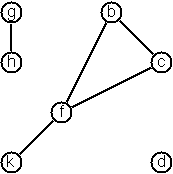A graph is defined as a set of nodes and a set of edges, where each edge is a pair of nodes.

The class to represent a graph is mutable, which isn't in keeping with pure functional programming, but a pure functional data structure would make things much, much more complicated. [Pure functional graphs with cycles require laziness; I think Scala can handle it, but I think that would add too much of a barrier to the following questions. <PMG>]

Our `Graph`s use an incidence list internally. Each has a list of nodes and a list of edges. Each node also has a list of edges that connect it to other nodes. In a directed graph, nodes that are the target of arcs do not have references to those arcs in their adjacency list.

```abstract class GraphBase[T, U] {
case class Edge(n1: Node, n2: Node, value: U) {
def toTuple = (n1.value, n2.value, value)
}
case class Node(value: T) {
def neighbors: List[Node] = adj.map(edgeTarget(_, this).get)
}

var nodes: Map[T, Node] = Map()
var edges: List[Edge] = Nil

// If the edge E connects N to another node, returns the other node,
// otherwise returns None.
def edgeTarget(e: Edge, n: Node): Option[Node]

override def equals(o: Any) = o match {
case g: GraphBase[T,U] => (nodes.keys.toList -- g.nodes.keys.toList == Nil &&
edges.map(_.toTuple) -- g.edges.map(_.toTuple) == Nil)
case _ => false
}

val n = new Node(value)
nodes = Map(value -> n) ++ nodes
n
}
}

class Graph[T, U] extends GraphBase[T, U] {
override def equals(o: Any) = o match {
case g: Graph[T,U] => super.equals(g)
case _ => false
}

def edgeTarget(e: Edge, n: Node): Option[Node] =
if (e.n1 == n) Some(e.n2)
else if (e.n2 == n) Some(e.n1)
else None

def addEdge(n1: T, n2: T, value: U) = {
val e = new Edge(nodes(n1), nodes(n2), value)
edges = e :: edges
}
}

class Digraph[T, U] extends GraphBase[T, U] {
override def equals(o: Any) = o match {
case g: Digraph[T,U] => super.equals(g)
case _ => false
}

def edgeTarget(e: Edge, n: Node): Option[Node] =
if (e.n1 == n) Some(e.n2)
else None

def addArc(source: T, dest: T, value: U) = {
val e = new Edge(nodes(source), nodes(dest), value)
edges = e :: edges
}
}```

The full initial Graph code, which also includes objects for creating graphs, is in graph1.scala.

There are a few ways to create a graph from primitives. The graph-term form lists the nodes and edges separately:

```Graph.term(List('b', 'c', 'd', 'f', 'g', 'h', 'k'),
List(('b', 'c'), ('b', 'f'), ('c', 'f'), ('f', 'k'), ('g', 'h')))```

The adjacency-list form associates each node with its adjacent nodes. In an undirected graph, care must be taken to ensure that all links are symmetric--if b is adjacent to c, c must also be adjacent to b.

```Graph.adjacent(List(('b', List('c', 'f')), ('c', List('b', 'f')), ('d', Nil),
('f', List('b', 'c', 'k')), ('g', List('h')), ('h', List('g')),
('k', List('f'))))```

The representations we introduced so far are bound to our implementation and therefore well suited for automated processing, but their syntax is not very user-friendly. Typing the terms by hand is cumbersome and error-prone. We can define a more compact and "human-friendly" notation as follows: A graph is represented by a string of terms of the type X or Y-Z separated by commas. The standalone terms stand for isolated nodes, the Y-Z terms describe edges. If an X appears as an endpoint of an edge, it is automatically defined as a node. Our example could be written as:

`[b-c, f-c, g-h, d, f-b, k-f, h-g]`

We call this the human-friendly form. As the example shows, the list does not have to be sorted and may even contain the same edge multiple times. Notice the isolated node d.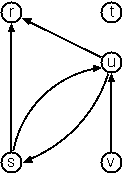When the edges of a graph are directed, we call them arcs. These are represented by ordered pairs. Such a graph is called directed graph. To represent a directed graph, the forms discussed above are slightly modified. The example graph opposite is represented as follows:

graph-term form:

```Digraph.term(List('r', 's', 't', 'u', 'v'),
List(('s', 'r'), ('s', 'u'), ('u', 'r'), ('u', 's'), ('v', 'u')))```

```Digraph.adjacent(List(('r', Nil), ('s', List('r', 'u')), ('t', Nil),
('u', List('r', 's')), ('v', List('u'))))```

(Note that the adjacency-list form is the same for graphs and digraphs.)

human-friendly form:

`[s>r, t, u>r, s>u, u>s, v>u]`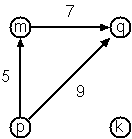Finally, graphs and digraphs may have additional information attached to nodes and edges (arcs). For the nodes, this is no problem, as we can put any type into them. On the other hand, for edges we have to extend our notation. Graphs with additional information attached to edges are called labeled graphs.

graph-term form:

```Digraph.termLabel(List('k', 'm', 'p', 'q'),
List(('m', 'q', 7), ('p', 'm', 5), ('p', 'q', 9)))```

```Digraph.adjacentLabel(
List(('k', Nil), ('m', List(('q', 7))), ('p', List(('m', 5), ('q', 9))),
('q', Nil)))```

human-friendly form:

`[p>q/9, m>q/7, k, p>m/5]`

The notation for labeled graphs can also be used for so-called multi-graphs, where more than one edge (or arc) is allowed between two given nodes.

P80 (***) Conversions.
Write methods to generate the graph-term and adjacency-list forms from a `Graph`. Write another method to output the human-friendly form for a graph. Make it the `toString` method for `Graph`. Write more functions to create graphs from strings.

Hint: You might need separate functions for labeled and unlabeled graphs.

```scala> Graph.fromString("[b-c, f-c, g-h, d, f-b, k-f, h-g]").toTermForm
res0: (List[String], List[(String, String, Unit)]) = (List(d, k, h, c, f, g, b),List((h,g,()), (k,f,()), (f,b,()), (g,h,()), (f,c,()), (b,c,())))

res1: List[(String, List[(String, Int)])] = List((m,List((q,7))), (p,List((m,5), (q,9))), (k,List()), (q,List()))```
P81 (**) Path from one node to another one.
Write a method named `findPaths` to find acyclic paths from one node to another in a graph. The method should return all paths.
```scala> Digraph.fromStringLabel("[p>q/9, m>q/7, k, p>m/5]").findPaths("p", "q")
res0: List[List[String]] = List(List(p, q), List(p, m, q))

scala> Digraph.fromStringLabel("[p>q/9, m>q/7, k, p>m/5]").findPaths("p", "k")
res1: List[List[String]] = List()```
P82 (*) Cycle from a given node.
Write a method named `findCycles` to find closed paths (cycles) starting at a given node in a graph. The method should return all cycles.
```scala> Graph.fromString("[b-c, f-c, g-h, d, f-b, k-f, h-g]").findCycles("f")
res0: List[List[String]] = List(List(f, c, b, f), List(f, b, c, f))```
P83 (**) Construct all spanning trees.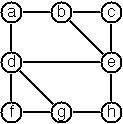Write a method `spanningTrees` to construct all spanning trees of a given graph. With this method, find out how many spanning trees there are for the graph depicted to the right. The data of this example graph can be found below. When you have a correct solution for the `spanningTrees` method, use it to define two other useful methods: `isTree` and `isConnected`. Both are five-minute tasks!

Graph:

```Graph.term(List('a', 'b', 'c', 'd', 'e', 'f', 'g', 'h'),
List(('a', 'b'), ('a', 'd'), ('b', 'c'), ('b', 'e'),
('c', 'e'), ('d', 'e'), ('d', 'f'), ('d', 'g'),
('e', 'h'), ('f', 'g'), ('g', 'h')))```
```scala> Graph.fromString("[a-b, b-c, a-c]").spanningTrees
res0: List[Graph[String,Unit]] = List([a-b, b-c], [a-c, b-c], [a-b, a-c])```
P84 (**) Construct the minimal spanning tree.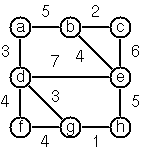Write a method `minimalSpanningTree` to construct the minimal spanning tree of a given labeled graph. Hint: Use Prim's Algorithm. A small modification of the solution of P83 does the trick. The data of the example graph to the right can be found below.

Graph:

```Graph.termLabel(
List('a', 'b', 'c', 'd', 'e', 'f', 'g', 'h'),
List(('a', 'b', 5), ('a', 'd', 3), ('b', 'c', 2), ('b', 'e', 4),
('c', 'e', 6), ('d', 'e', 7), ('d', 'f', 4), ('d', 'g', 3),
('e', 'h', 5), ('f', 'g', 4), ('g', 'h', 1)))```
```scala> Graph.fromStringLabel("[a-b/1, b-c/2, a-c/3]").minimalSpanningTree
res0: Graph[String,Int] = [a-b/1, b-c/2]```
P85 (**) Graph isomorphism.
Two graphs G1(N1,E1) and G2(N2,E2) are isomorphic if there is a bijection f: N1N2 such that for any nodes X,Y of N1, X and Y are adjacent if and only if f(X) and f(Y) are adjacent.

Write a method that determines whether two graphs are isomorphic.

```scala> Graph.fromString("[a-b]").isIsomorphicTo(Graph.fromString("[5-7]"))
res0: Boolean = true```
P86 (**) Node degree and graph coloration.
a) Write a method `Node.degree` that determines the degree of a given node.
```scala> Graph.fromString("[a-b, b-c, a-c, a-d]").nodes("a").degree
res0: Int = 3```

b) Write a method that lists all nodes of a graph sorted according to decreasing degree.

```scala> Graph.fromString("[a-b, b-c, a-c, a-d]").nodesByDegree
res1: List[Graph[String,Unit]#Node] = List(Node(a), Node(c), Node(b), Node(d))```

c) Use Welsh-Powell's algorithm to paint the nodes of a graph in such a way that adjacent nodes have different colors. Make a method `colorNodes` that returns a list of tuples, each of which contains a node and an integer representing its color.

```scala> Graph.fromString("[a-b, b-c, a-c, a-d]").colorNodes
res2: List[(Graph[String,Unit]#Node,Int)] = List((Node(a),1), (Node(b),2), (Node(c), 3), (Node(d), 2))```
P87 (**) Depth-first order graph traversal.
Write a method that generates a depth-first order graph traversal sequence. The starting point should be specified, and the output should be a list of nodes that are reachable from this starting point (in depth-first order).
```scala> Graph.fromString("[a-b, b-c, e, a-c, a-d]").nodesByDepthFrom("d")
res0: List[String] = List(c, b, a, d)```
P88 (**) Connected components.
Write a function that splits a graph into its connected components.
```scala> Graph.fromString("[a-b, c]").splitGraph
res0: List[Graph[String,Unit]] = List([a-b], [c])```
P89 (**) Bipartite graphs.
Write a function that determines whether a given graph is bipartite.
```scala> Digraph.fromString("[a>b, c>a, d>b]").isBipartite
res0: Boolean = true

scala> Graph.fromString("[a-b, b-c, c-a]").isBipartite
res1: Boolean = false

scala> Graph.fromString("[a-b, b-c, d]").isBipartite
res2: Boolean = true

scala> Graph.fromString("[a-b, b-c, d, e-f, f-g, g-e, h]").isBipartite
res3: Boolean = false```

The complete source file for this section is graph.scala.

## Miscellaneous Problems

P90 (**) Eight queens problem
This is a classical problem in computer science. The objective is to place eight queens on a chessboard so that no two queens are attacking each other; i.e., no two queens are in the same row, the same column, or on the same diagonal.

Hint: Represent the positions of the queens as a list of numbers 1..N. Example: `List(4, 2, 7, 3, 6, 8, 5, 1)` means that the queen in the first column is in row 4, the queen in the second column is in row 2, etc. Use the generate-and-test paradigm.

P91 (**) Knight's tour.
Another famous problem is this one: How can a knight jump on an N×N chessboard in such a way that it visits every square exactly once?

Hints: Represent the squares by pairs of their coordinates of the form (X, Y), where both X and Y are integers between 1 and N. (Alternately, define a Point class for the same purpose.) Write a function jumps(N, (X, Y)) to list the squares that a knight can jump to from (X, Y) on a N×N chessboard. And finally, represent the solution of our problem as a list of knight positions (the knight's tour).

It might be nice to find more than one tour, but a computer will take a long time trying to find them all at once. Can you make a lazy list that only calculates the tours as needed?

Can you find only "closed tours", where the knight can jump from its final position back to its starting position?

P92 (***) Von Koch's conjecture.
Several years ago I met a mathematician who was intrigued by a problem for which he didn't know a solution. His name was Von Koch, and I don't know whether the problem has been solved since. [The "I" here refers to the author of the Prolog problems. <PMG>]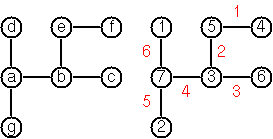Anyway the puzzle goes like this: Given a tree with N nodes (and hence N-1 edges), find a way to enumerate the nodes from 1 to N and, accordingly, the edges from 1 to N-1 in such a way, that for each edge K the difference of its node numbers is equal to K. The conjecture is that this is always possible.

For small trees the problem is easy to solve by hand. However, for larger trees, and 14 is already very large, it is extremely difficult to find a solution. And remember, we don't know for sure whether there is always a solution!

Write a function that calculates a numbering scheme for a given tree. What is the solution for the larger tree pictured below?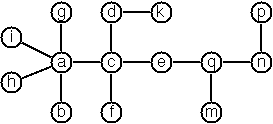P93 (***) An arithmetic puzzle.
Given a list of integer numbers, find a correct way of inserting arithmetic signs (operators) such that the result is a correct equation. Example: With the list of numbers `List(2,3,5,7,11)` we can form the equations 2-3+5+7 = 11 or 2 = (3*5+7)/11 (and ten others!).

P94 (***) Generate K-regular simple graphs with N nodes.
In a K-regular graph all nodes have a degree of K; i.e. the number of edges incident in each node is K. How many (non-isomorphic!) 3-regular graphs with 6 nodes are there? See also a table of results and a Java applet that can represent graphs geometrically.

P95 (**) English number words.
On financial documents, like checks, numbers must sometimes be written in full words. Example: 175 must be written as one-seven-five. Write a function fullWords(num: Int) to print (non-negative) integer numbers in full words.

P96 (**) Syntax checker.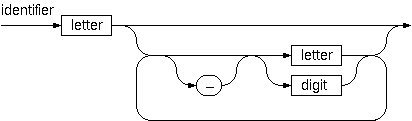In a certain programming language (Ada) identifiers are defined by the syntax diagram (railroad chart) opposite. Transform the syntax diagram into a system of syntax diagrams which do not contain loops; i.e. which are purely recursive. Using these modified diagrams, write a function isIdentifier that can check whether or not a given string is a legal identifier.

P97 (**) Sudoku. (alternate solution)
Sudoku puzzles go like this:
```   Problem statement                 Solution

.  .  4 | 8  .  . | .  1  7	     9  3  4 | 8  2  5 | 6  1  7
|         |                      |         |
6  7  . | 9  .  . | .  .  .	     6  7  2 | 9  1  4 | 8  5  3
|         |                      |         |
5  .  8 | .  3  . | .  .  4      5  1  8 | 6  3  7 | 9  2  4
--------+---------+--------      --------+---------+--------
3  .  . | 7  4  . | 1  .  .      3  2  5 | 7  4  8 | 1  6  9
|         |                      |         |
.  6  9 | .  .  . | 7  8  .      4  6  9 | 1  5  3 | 7  8  2
|         |                      |         |
.  .  1 | .  6  9 | .  .  5      7  8  1 | 2  6  9 | 4  3  5
--------+---------+--------      --------+---------+--------
1  .  . | .  8  . | 3  .  6	     1  9  7 | 5  8  2 | 3  4  6
|         |                      |         |
.  .  . | .  .  6 | .  9  1	     8  5  3 | 4  7  6 | 2  9  1
|         |                      |         |
2  4  . | .  .  1 | 5  .  .      2  4  6 | 3  9  1 | 5  7  8```

Every spot in the puzzle belongs to a (horizontal) row and a (vertical) column, as well as to one single 3×3 square (which we call "square" for short). At the beginning, some of the spots carry a single-digit number between 1 and 9. The problem is to fill the missing spots with digits in such a way that every number between 1 and 9 appears exactly once in each row, in each column, and in each square.

P98 (***) Nonograms.
Around 1994, a certain kind of puzzles was very popular in England. The "Sunday Telegraph" newspaper wrote: "Nonograms are puzzles from Japan and are currently published each week only in The Sunday Telegraph. Simply use your logic and skill to complete the grid and reveal a picture or diagram." As a programmer, you are in a better situation: you can have your computer do the work! Just write a little program ;-).

The puzzle goes like this: Essentially, each row and column of a rectangular bitmap is annotated with the respective lengths of its distinct strings of occupied cells. The person who solves the puzzle must complete the bitmap given only these lengths.

```          Problem statement:          Solution:

|_|_|_|_|_|_|_|_| 3         |_|X|X|X|_|_|_|_| 3
|_|_|_|_|_|_|_|_| 2 1       |X|X|_|X|_|_|_|_| 2 1
|_|_|_|_|_|_|_|_| 3 2       |_|X|X|X|_|_|X|X| 3 2
|_|_|_|_|_|_|_|_| 2 2       |_|_|X|X|_|_|X|X| 2 2
|_|_|_|_|_|_|_|_| 6         |_|_|X|X|X|X|X|X| 6
|_|_|_|_|_|_|_|_| 1 5       |X|_|X|X|X|X|X|_| 1 5
|_|_|_|_|_|_|_|_| 6         |X|X|X|X|X|X|_|_| 6
|_|_|_|_|_|_|_|_| 1         |_|_|_|_|X|_|_|_| 1
|_|_|_|_|_|_|_|_| 2         |_|_|_|X|X|_|_|_| 2
1 3 1 7 5 3 4 3             1 3 1 7 5 3 4 3
2 1 5 1                     2 1 5 1                      ```

For the example above, the problem can be stated as the two lists `[,[2,1],[3,2],[2,2],,[1,5],,,]` and `[[1,2],[3,1],[1,5],[7,1],,,,]` which give the "solid" lengths of the rows and columns, top-to-bottom and left-to-right, respectively. Published puzzles are larger than this example, e.g. 25×20, and apparently always have unique solutions.

P99 (***) Crossword puzzle.
Given an empty (or almost empty) framework of a crossword puzzle and a set of words. The problem is to place the words into the framework.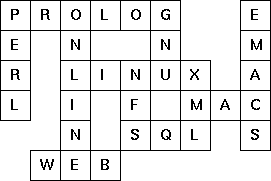The particular crossword puzzle is specified in a text file which first lists the words (one word per line) in an arbitrary order. Then, after an empty line, the crossword framework is defined. In this framework specification, an empty character location is represented by a dot (.). In order to make the solution easier, character locations can also contain predefined character values. The puzzle opposite is defined in the file p99a.dat, other examples are p99b.dat and p99d.dat. There is also an example of a puzzle (p99c.dat) which does not have a solution.

Words are strings of at least two characters. A horizontal or vertical sequence of character places in the crossword puzzle framework is called a site. Our problem is to find a compatible way of placing words onto sites.

Hints: (1) The problem is not easy. You will need some time to thoroughly understand it. So, don't give up too early! And remember that the objective is a clean solution, not just a quick-and-dirty hack!

(2) For efficiency reasons it is important, at least for larger puzzles, to sort the words and the sites in a particular order. For this part of the problem, the solution of P28 may be very helpful.